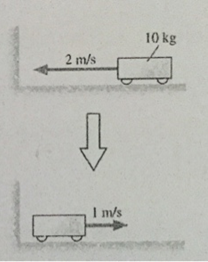# Problem: The cart’s change of momentum Δpx isA. -20 kg m/sB.-10 kg m/sC. 0 kg m/sD. 10 kg m/sE. 30 kg m/s

###### FREE Expert Solution

Momentum:

$\overline{){\mathbf{p}}{\mathbf{=}}{\mathbf{m}}{\mathbf{v}}}$

Initial momentum, pi = (10)(-2) = -20 kg•m/s

91% (472 ratings)###### Problem DetailsThe cart’s change of momentum Δpx is

A. -20 kg m/s

B.-10 kg m/s

C. 0 kg m/s

D. 10 kg m/s

E. 30 kg m/s